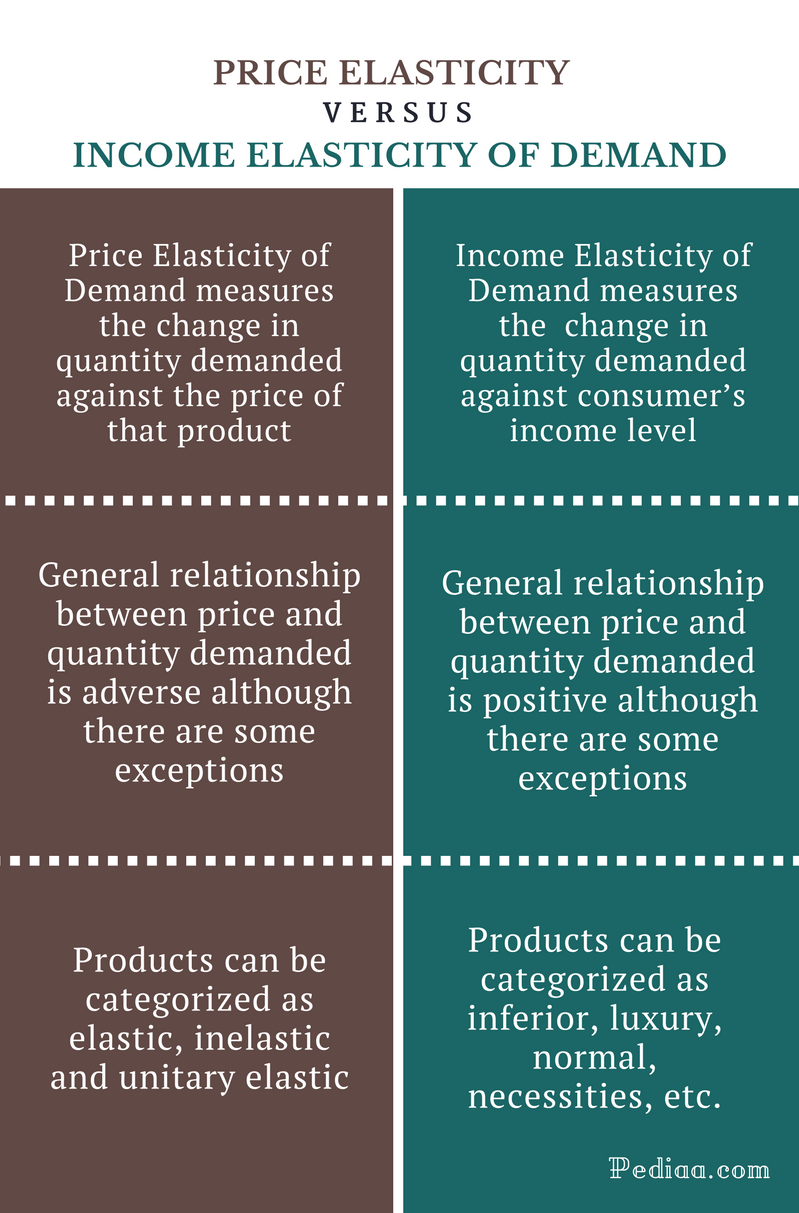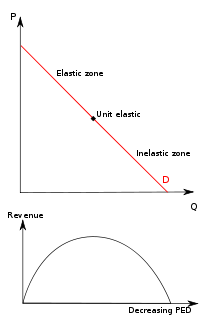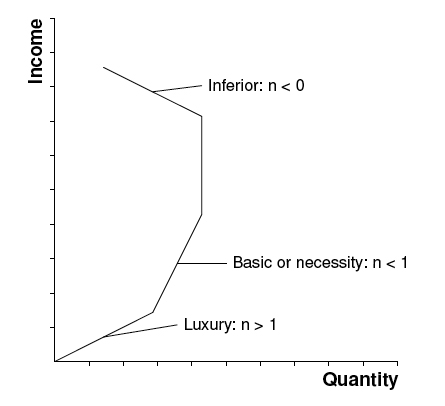# Distinguish Between Price Elasticity and Income Elasticity of Demand

## Main Difference  – Price Elasticity vs Income Elasticity of Demand

Elasticity is a common measure widely used in Economics pertaining to different parameters such as price, income, prices of associated goods and services. Elasticity can be defined as a measure of the responsiveness of quantity (demand / supply) as a result of a change in the independent variables such as the change in the price of goods and change in consumer income in the market. Price elasticity of demand is the degree of responsiveness of quantity demanded, with respect to the market price changes. Income elasticity of demand measures the responsiveness of the quantity demanded, with respect to the change in consumer’s income. Knowing this difference will help you to distinguish between price elasticity and income elasticity of demand.

1. What is Price Elasticity of Demand? – Definition, Formula for Calculation, Determinants, etc.

2. What is Income Elasticity of Demand? – Definition, Formula for Calculation, etc.

3. How to Distinguish Between Price Elasticity and Income Elasticity of Demand## What is Price Elasticity of Demand

Price elasticity of demand can be simply defined as the degree of responsiveness of quantity demanded with respect to the market price changes. The formula to calculate price elasticity of demand is,

PED = (Percentage change in quantity demanded / Percentage change in price)

There are few determinants of price elasticity of demand.

• Availability of substitute products – If there are many substitutes available in the market for a particular good, people have different choices among them. Then they will highly respond to the price changes.
• Proportion of the consumer’s income – If the proportion is high, elasticity is high
• Time period – In long run price elasticity of demand in high
• Necessity – If the good is essential, people are less responsive to the price changes

In a general context, there’s a negative relationship between price and quantity demanded of a particular good. That is when the price of a product increases, customer’s purchasing ability reduces resulting in lower demand.## What is Income Elasticity of Demand

The income elasticity of demand measures the responsiveness of the quantity demanded, with respect to the change in consumer’s income. This can be calculated by the following formula.

PED = (Percentage change in quantity demanded / Percentage change in consumer income)

In a general context, there’s a positive relationship between price and quantity demanded of a particular good. That is, when the consumer income increases, people will demand more goods from the marketplace.## Similarities Between Price Elasticity and Income Elasticity of Demand

• Both are measures of the responsiveness of quantity demanded by the consumers in a given economy, only the underlying reason is the change.

## How to Distinguish Between Price Elasticity and Income Elasticity of Demand

### What it Measures

Price Elasticity of Demand: Price Elasticity of Demand measures the change in quantity demanded against the price of that product.

Income Elasticity of Demand: Income Elasticity of Demand measures the  change in quantity demanded against consumer’s income level.

### Price vs Quantity

Price Elasticity of Demand: The general relationship between price and quantity demanded is adverse although there are some exceptions.

Income Elasticity of Demand: The general relationship between price and quantity demanded is positive although there are some exceptions.

### Application

Price Elasticity of Demand: Based on the coefficient of price elasticity of demand calculation; products can be categorized as elastic, inelastic and unitary elastic.

Income Elasticity of Demand: Based on the coefficient of price elasticity of demand calculation, products can be categorized as inferior, luxury, normal, necessities, etc.

## Price Elasticity vs Income Elasticity of Demand – Conclusion

Price elasticity of demand and income elasticity of demand are two important calculations in economics. Price elasticity of demand measures the responsiveness of quantity demanded of a particular product as a result of a change in price levels. In contrast, the income elasticity of demand measures the responsiveness of quantity demanded as a result of a change in consumer’s income levels. In general, the price elasticity of demand is a negative figure, whilst income elasticity is a positive figure although there are some exceptional situations that change the above general laws. These elasticity concepts provide higher importance in making several economic decisions such as international trade, factor pricing (rent, wages, interest, and profits), formulation of government policies, etc.

Image Courtesy:

“Price elasticity of demand and revenue” By Price_elasticity_of_demand_and_revenue.png: Original uploader was RedWordSmith at en.wikipediaderivative work: Jarry1250 (talk) (CC BY-SA 3.0) via Commons Wikimedia

“Income elasticity” By various – review docs (Public Domain) via Commons Wikimedia